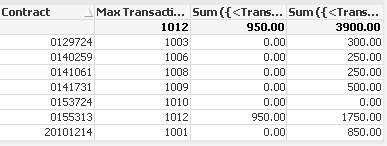# QlikView Creating Analytics

Discussion Board for collaboration related to Creating Analytics for QlikView.

HighlightedNot applicable

## Help with Set Analysis

Hi Community:

I have some issues calculating in set analysis.

This is my data. I have many transacionID per contract. What I'm trying to do is to get the Amount on the Max of TransactionID, within the date range (date entered by an user) and when ObjectID = 615. So the red boxes are the Max TransactionID between 10/1/2011 and 9/30/2012.I have calculated the Max of the TransactionID like this:

Max({<Date={'>=\$(vStartDate)<=\$(vEndDate)'}>}TransactionID)

This works fine, but then I want to get the Amount including the above set analysis and does not work.I have tried 2 options:

1. This calculation give me the results only when clicking a Contract, and that is not what I'm looking for. I want to always display the values

Sum ({<TransactionID={\$(=Max({<Date={'>=\$(vStartDate)<=\$(vEndDate)'}>}TransactionID))}, ObjectID={615}>}Amount)

2. This gives me something else but not the solution

Sum ({<TransactionID={"=Max({<Date={'>=\$(vStartDate)<=\$(vEndDate)'}>}TransactionID)"}, ObjectID={615}>}distinct Amount)

The expected resultd should be:

 Contract Amount 20101214 450.00 0129724 0.00 0140259 100.00 0141061 250.00 0141731 500.00 0153724 0.00 0155313 950.00

//0129724 is 0 because does not satisfy the condition of Obj=615 on the Max. Transaction ID which is 1003

Any ideas? Attached are my files.

Thanks!

Tags (8)
1 Solution

Accepted SolutionsMVP

## Re: Help with Set Analysis

Try

=FirstSortedValue( {<Date={">=\$(vStartDate)<=\$(vEndDate)"}>}

aggr(sum({< ObjectID={615}>} Amount),TransactionID),

-TransactionID )

)

7 RepliesPartner

## Re: Help with Set Analysis

Try this:

Sum ({<TransactionID={\$(=Concat(aggr(max(TransactionID), Contract), ', '))}, Date={">=\$(vStartDate)<=\$(vEndDate)"}, ObjectID={615}>}Amount)MVP & Luminary

## Re: Help with Set Analysis

Hi,

Please find the file attached.

Regards,

Jagan.Not applicable

## Re: Help with Set Analysis

Thanks Fernado. The expression works, but the issue is my data set is huge. I have millions of transactions and the "Concat" function is taking forever to get all the values for a specific date.Not applicable

## Re: Help with Set Analysis

Any other idea without using the "Concat" function?MVP

## Re: Help with Set Analysis

Try

=FirstSortedValue( {<Date={">=\$(vStartDate)<=\$(vEndDate)"}>}

aggr(sum({< ObjectID={615}>} Amount),TransactionID),

-TransactionID )

)Not applicable

## Re: Help with Set Analysis

Thanks swuehl!! This worked really good. Could you explain please how the FirstSortedValue function worked for this case?Not applicable

## Re: Help with Set Analysis

Can you try this?  I just want to see the performance difference.

I think this should reduce the amount of data in Concat() portion.

sum({<TransactionID={\$(=concat(aggr(Max({<Date={">=\$(vStartDate)<=\$(vEndDate)"}>}TransactionID), Contract),', '))}, ObjectID={615}>}Amount)

Sean